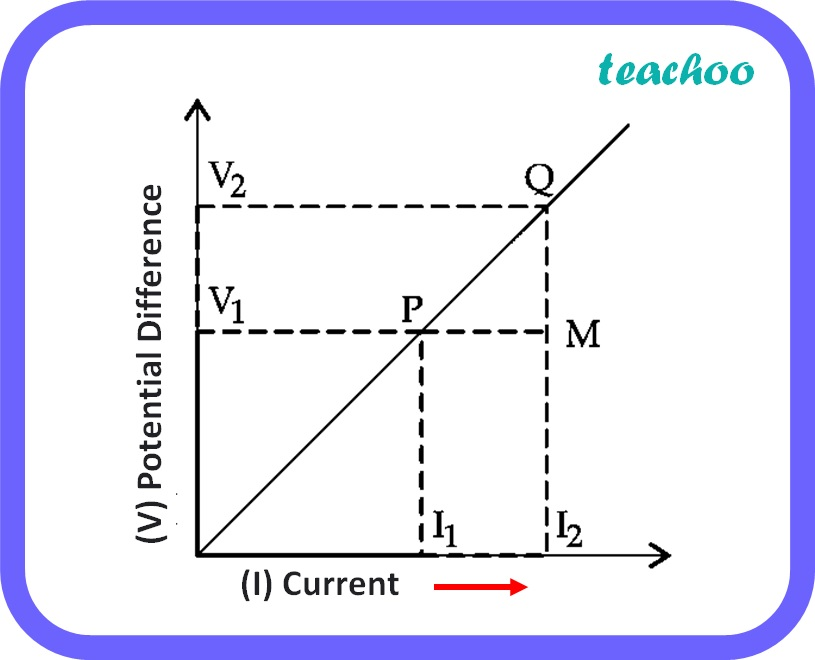Past Year - 2 Mark Questions

Class 10
Chapter 12 Class 10 - Electricity

## While studying the dependence of potential difference (V) across a resistor on the current (I) passing through it, in order to determine the resistance of the resistor, a student took 5 readings for different values of current and plotted a graph between V and I. He got a straight line graph passing through the origin. What does the straight line signify? Write the method of determining resistance of the resistor using this graph.

The graph between V and I is a straight line graph which passes through the origin.The straight line signifies that potential difference (V) is directly proportional to Current (I).

The slope of the straight line gives the resistance of the resistor.

Resistance or Slope = ( V 2 - V 1 ) / ( I 2 - I 1 )

Learn in your speed, with individual attention - Teachoo Maths 1-on-1 Class